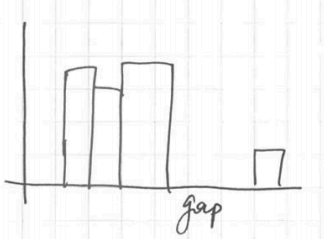# ML Wiki

## The Shape of Data

Distribution - the pattern of values in the data, showing their frequency of occurrence relative to each other.

## Plots

There are some plots that can be useful for showing the distribution of data

### Histograms

Histogram is useful to show distribution of data

• Bins: the intervals used in a histogram. The data must be separated into mutually exclusive and exhaustive bins
• Cutpoints: the values that define the beginning and the end of the bins
• Frequency: the count of the number of the data values in each bin
• The peaks in the distribution are called modes

We can group distributions according to the number of modes they have:

• unimodal - a distribution with one mode
• bimodal - with 2 peaks
• multimodal - more than 2 peaks

In R:

hist(..., breaks=10, ...) // histogram


### Density Plots

Like a histogram, but smoothed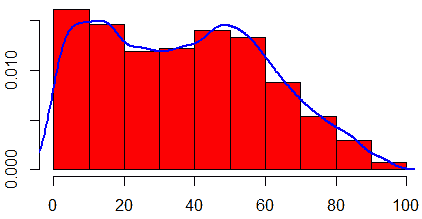## Types

There are many distributions:

• Uniform Distribution - equally spread without any mode
• symmetric
• the mean, median, and mode are all approximately the same.
•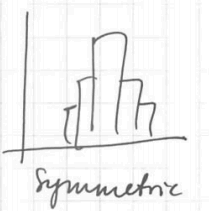• assymetric
•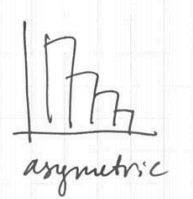• left-skewed
• the longer tail on the left side
• the mode is larger than the median which is larger than the mean
• right-skewed
• the longer tail on the right side
• the mode is less than the median which is less the mean
•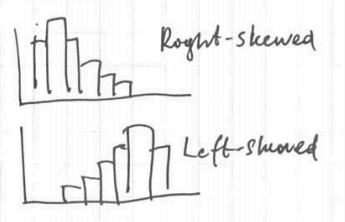• with gap
•